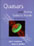© CAMBRIDGE UNIVERSITY PRESS 1999

1.3.2 Gravitational redshift

Einstein's general theory of relativity drew the attention of astronomers to this source of redshift: indeed, the early demonstrations of this effect were for the white dwarf stars Sirius B andEridani B. In either case the effect was very small, being less than 0.001. Theoretically one could, on the basis of Schwarzschild's solution, define the surface redshift of a mass M with radius R by the formula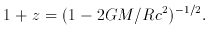(1.3)

Thus, by having R arbitrarily close to the critical value 2GM / c2, it may seem possible to have large redshifts. This is not, however, the case.

In 1964 Hermann Bondi showed that whatever equation of state is chosen, provided it is physically realistic (i.e., with sound speed in the material not exceeding the speed of light), the surface redshift cannot exceed 0.62. Thus surface gravitational redshift proved inadequate to explain the large redshifts of quasars. There was also an observational objection put forward by J. Greenstein and M. Schmidt (1964). We briefly reproduce their elegant argument as applied to the quasar 3C 273.

For small redshifts we get from the above formula(1.4)

Consider a thin shell of radius R and thicknessR emitting radiation in a line of width w. For line widths in 3C 273 arising from the slight change of redshift overR we get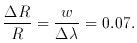(1.5)

Now suppose that the object is of stellar type with mass ~ M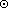. For such an object a redshift 0.158 as in 3C 273 gives a radius of ~ 106 cm, and soR ~ 7 x 104 cm.

Next we determine the volume of the shell and use the volume emissivity of the Hline to estimate the flux of radiation in that line. For the source 3C 273 the volume emissivity at a temperature ~ 104 K is ~ 10-25 ne2 erg sec-1 cm-3, where ne is the electron number density. Multiplying this with the volume of the shell and equating the result to the observed luminosity we get(1.6)

where d is the expected distance of the source measured in centimetres. This gives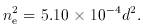(1.7)

Since the searches for proper motion of 3C 273 proved negative, Greenstein and Schmidt assumed that the source could not be nearer than 100 pc. This gave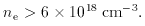(1.8)

This density is too high, and is precluded by the appearance of the forbidden line [O III]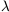5007 in the source spectrum. Thus the stellar possibility is ruled out. Similarly Greenstein and Schmidt were able to reject the alternative hypothesis that the source is much more massive, extragalactic and located within, say, 25 Mpc.

To get round this argument and the Bondi limit, Hoyle and Fowler (1967) proposed a rather unusual model of a quasar in which the redshift arose from light coming from the interior. In this model the gravitational potential well at the centre of the object is provided by a distribution of a large number of very compact objects (such as, say, neutron stars). Any gas present in the system would descend to the centre and settle down there and form the emission line region. It is not difficult to see that the gravitating compact objects would leave a sufficient gap for the central radiation to emerge with redshifts much higher than the Bondi limit. The interior Schwarzschild solution used by Hoyle and Fowler to demonstrate this effect gives arbitrarily high central redshifts. However, more realistic models discussed by P.K. Das (1977) yielded redshifts of the order of 2-3. These models also get around the Greenstein-Schmidt criticism provided the masses are of galactic order.

We will take a second look at the gravitational redshift option in Chapter 15, when considering other evidence that has a bearing on the nature of redshifts.